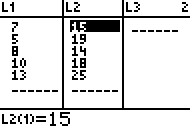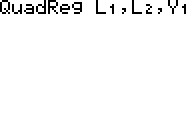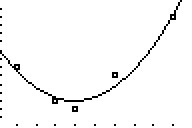# Knowledge Base

## Solution 11931: Calculating and Graphing Quadratic Regressions Using the TI-83 Family, TI-84 Plus Family Graphing Calculator, and TI-Nspire™ Handheld in TI-84 Plus Mode.

### How do I calculate and graph a quadratic regression using the TI-83 family, TI-84 Plus family, and TI-Nspire handheld in TI-84 Plus mode?

The example below will demonstrate how to calculate and graph a quadratic regression using the TI-83 family, TI-84 Plus family, and TI-Nspire handheld in TI-84 Plus mode.

Data for this example:To enter the data:
1) Press [STAT]  to access the STAT list editor.
2) Input the data in the L1 and L2 lists, pressing [ENTER] after each number.
3) Press [2nd] [MODE] to QUIT and return to the home screen.

To calculate the quadratic regression (ax2+bx+c):
4) Press [STAT] to access the STAT menu.
5) Scroll right to highlight the CALC menu.
6) Press  to select QuadReg(ax+b).
7) Press [2nd]  [,] [2nd]  to input L1,L2.
8) Press [,] [VARS], scroll right to highlight Y-VARS, then press   to input ,Y1.
9) Press [ENTER] to calculate the quadratic regression. This will also copy the quadratic regression equation to the Y= Editor.To graph the data and the quadratic regression equation:
10) Press [2nd] [Y=]  to access the STAT PLOTS menu and edit Plot1.
11) Press [ENTER] to turn On Plot1.
12) Scroll down to Type: and press [ENTER] to select the scatter plot option.
13) Scroll down to Xlist and press [2nd]  to input L1. Scroll down to Ylist and press [2nd]  to input L2.14) Press [GRAPH] to graph the data and the quadratic regression equation.
15) If the graphs are not displayed, press [ZOOM]  to perform a ZoomStat.Please see the TI-83 Plus and TI-84 Plus guidebooks for additional information.

TI-Nspire handheld in TI-84 Plus mode users may refer to the TI-84 Plus guidebook.